the arc of software bends towards understanding

## You could have invented fractional cascading

Suppose that you have k sorted arrays, each of size n. You would like to search for single element in each of the k arrays (or its predecessor, if it doesn't exist).Obviously you can binary search each array individually, resulting in a$O(k\lg n)$ runtime. But we might think we can do better that: after all, we're doing the same search k times, and maybe we can "reuse" the results of the first search for later searches.

Here's another obvious thing we can do: for every element in the first array, let's give it a pointer to the element with the same value in the second array (or if the value doesn't exist, the predecessor.) Then once we've found the item in the first array, we can just follow these pointers down in order to figure out where the item is in all the other arrays.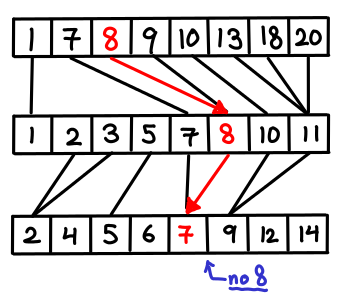But there's a problem: sometimes, these pointers won't help us at all. In particular, if a later lists is completely "in between" two elements of the first list, we have to redo the entire search, since the pointer gave us no information that we didn't already know.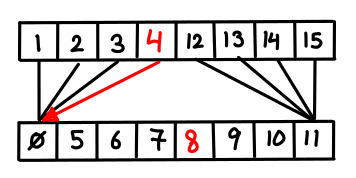So what do we do? Consider the case where k = 2; everything would be better if only we could guarantee that the first list contained the right elements to give you useful information about the second array. We could just merge the arrays, but if we did this in the general case we'd end up with a totally merged array of size$kn$, which is not so good if k is large.

But we don't need all of the elements of the second array; every other item will do!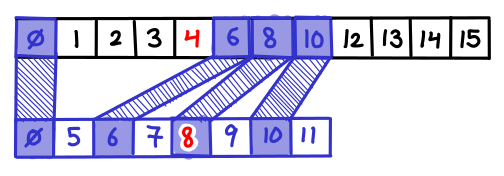Let's repeatedly do this. Take the last array, take every other element and merge it into the second to last array. Now, with the new second to last array, do this to the next array. Rinse and repeat. How big does the first array end up being? You can solve the recurrence:$T(k) = n + T(k-1)/2$, which is the geometric series$n + n/2 + n/4 + n/8 + \ldots = 2n$. Amazingly, the new first list is only twice as large, which is only one extra step in the binary search!What we have just implemented is fractional cascading! A fraction of any array cascades up the rest of the arrays.

There is one more detail which has to be attended to. When I follow a pointer down, I might end up on an element which is not actually a member of the current array (it was one that was cascaded up). I need to be able to efficiently find the next element which is a member of the current array (and there might be many cascaded elements jammed between it and the next member element, so doing a left-scan could take a long time); so for every cascaded element I store a pointer to the predecessor member element.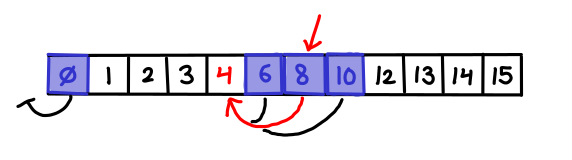Fractional cascading is a very useful transformation, used in a variety of contexts including layered range trees and 3D orthogonal range searching. In fact, it can be generalized in several ways. The first is that we can cascade some fixed fraction α of elements, rather than the 1/2 we did here. Additionally, we don't have to limit ourselves to cascading up a list of arrays; we can cascade up an arbitrary graph, merging many lists together as long as we pick α to be less than 1/d, where d is the in-degree of the node.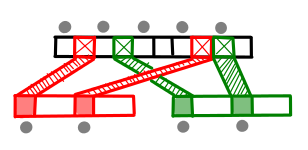Exercise. Previously, we described range trees. How can fractional cascading be used to reduce the query complexity by a factor of$O(\lg n)$?

Exercise. There is actually another way we can setup the pointers in a fractionally cascaded data structure. Rather than have downward pointers for every element, we only maintain pointers between elements which are identical (that is to say, they were cascaded up.) This turns out to be more convenient when you are constructing the data structure. However, you now need to maintain another set of pointers. What are they? (Hint: Consider the case where a search lands on a non-cascaded, member element.)

### 21 Responses to “You could have invented fractional cascading”

1.dankane says:

How is this any better than just merging all of the arrays and for each element of the big thing having a lookup table for the k answers? This seems like it would take basically the same amount of space and time (perhaps up to constants), require only log(nk) queries rather than log(n) + k queries, and be easier to implement.

2.Edward Z. Yang says:

That requires an extra O(nk^2) space for the lookup tables, if I understand correctly.

3.Andrew Pennebaker says:

If you have k sorted arrays, you could pretend they’re working arrays from a mergesort call upstream. Merging them together is O(kn), then binary searching the merged arrays is O(ln kn).

4.Edward Z. Yang says:

Yes, but one crucial difference is that you need to turn that single search into k elements, which as remarked above may require you to store lots of extra info!

5.dankane says:

OK. Fine. I can save on space by storing my lookup table as an array of pointers into my original array. Then I get by with O(nk log(nk)) space which is comparable unless k >> n.

6.dankane says:

Wait. Nevermind.

7.Kannan says:

What about storing one big array of (key,value) pairs sorted by the key, including duplicate keys. Once you binary search and find a matching key, just slide left/right to find all the other matching elements.

Sorry to bring this up again, but I still don’t understand why the single array technique doesn’t work as well (for the first use case, at least).

8.Edward Z. Yang says:

Kannan, the “slides” can take up to O(kn) time; just consider the case where you have arrays [1, 1, 1…], [2, 2, 2…], [3, 3, 3…], …

9.Kannan says:

In your example, what’s the exact result if we’re trying to search for the element “2”?

10.Edward Z. Yang says:

In the first list, the predecessor of 2 is 1, so we return 1. The second list is as you might expect. For all the other lists, we say no predecessor exists.

11.Kannan says:

Ah. I understand now. I forgot about having to return the closest predecessor element. Thanks!

12.Dave says:

13.Edward Z. Yang says:

Certainly it would have had to have been a good day!

14.Dhruv says:

Any idea how I can update (edit, insert, delete) values to/from the individual arrays in < O(n) time?

15.Dhruv says:

It would be super if you could post some problems (puzzles??) that require fractional cascading to solve them since an application of the technique would really nail the idea.

Thanks again!

16.Edward Z. Yang says:

Dhruv: This might be a reasonable starting point for dynamic updates: http://en.wikipedia.org/wiki/Fractional_cascading#Dynamic_fractional_cascading (you should also chase up the original paper).

As for application, I highly recommend you try out the first exercise. Check Demaine’s notes if you get stuck.

17.Dhruv says:

> As for application, I highly recommend you try out the first exercise. Check Demaine’s notes if you get stuck.

Thanks Edward! That’s a great idea!

18.Adito says:

Especially the using of the image.
Are you draw all of this manually?

19.Edward Z. Yang says:

21.Anonymous says: